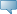# Lebanese American University WWW Information System

## Catalog Entries

Date
Sep 22, 2023Select the Course Number to get further detail on the course. Select the desired Schedule Type to find available classes for the course.

 MTH 303 - Numerical Methods This course compares and contrasts various numerical analysis techniques, in addition to error definition, stability, the machine precision concepts, inexactness of computational approximations, the design, code, test, and debug programs that implement numerical methods, floating-point arithmetic, convergence, iterative solutions for finding roots (Newton’s Method), curve fitting, function approximation, numerical differentiation and integration, explicit and implicit methods, differential equations (Euler’s Method), and finite differences. Course Learning Outcomes: 1) Students will acquire the skills needed to approximate roots of nonlinear equations. 2) Students will be able to find polynomial interpolants using the Lagrange interpolation method and Newton’s divided difference. 3) Students will acquire the skills needed to approximate derivatives and definite integrals. 4) Students will acquire the skills needed to solve systems of linear equations and perform matrix operations. 3.000 Credit hours 3.000 Lecture hours Levels: Undergraduate Schedule Types: Lecture, Tutorial Computer Science & Mathematics Department